Download Presentation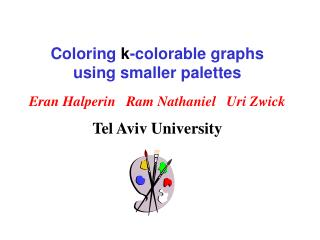Coloring k -colorable graphs using smaller palettes

# Coloring k -colorable graphs using smaller palettes - PowerPoint PPT Presentation

Coloring k -colorable graphs using smaller palettes. Eran Halperin Ram Nathaniel Uri Zwick Tel Aviv University. New coloring results. Coloring k -colorable graphs of maximum degree D using D 1 -2/k log 1/k D colors (instead of D 1 -2/k log 1/2 D colors [KMS] ).I am the owner, or an agent authorized to act on behalf of the owner, of the copyrighted work described.
Download Presentation## Coloring k -colorable graphs using smaller palettes

An Image/Link below is provided (as is) to download presentation

Download Policy: Content on the Website is provided to you AS IS for your information and personal use and may not be sold / licensed / shared on other websites without getting consent from its author.While downloading, if for some reason you are not able to download a presentation, the publisher may have deleted the file from their server.

- - - - - - - - - - - - - - - - - - - - - - - - - - E N D - - - - - - - - - - - - - - - - - - - - - - - - - -
Presentation Transcript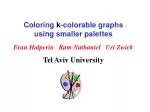### Coloring k-colorable graphsusing smaller palettes

Eran Halperin Ram Nathaniel Uri Zwick

Tel Aviv University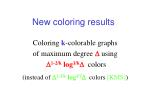### New coloring results

Coloring k-colorable graphs

of maximum degree Dusing

D1-2/klog1/kDcolors

(instead of D1-2/klog1/2Dcolors [KMS])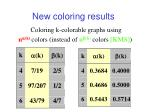### New coloring results

Coloring k-colorable graphs using

na(k)colors (instead of nb(k)colors [KMS])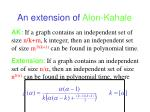### An extension of Alon-Kahale

AK: If a graph contains an independent set of size n/k+m, k integer, then an independent set of size m3/(k+1) can be found in polynomial time.

Extension: If a graph contains an independent set of size n/a, then an independent set of size nf(a) can be found in polynomial time, where### Graph coloring basics

If in any k-colorable graph on n vertices we can find, in polynomial time, one of

Two vertices that have the same color under some valid k-coloring ;

An independent set of size W(n1-a) ;

then we can color any k-colorable graph using O(na) colors.### Coloring 3-colorable graphs using O(n1/2) colors [Wigderson]

A graph with maximum degree D can be easily colored using D+1colors.

If D <n1/2, color using D+1colors.

Otherwise, let v be a vertex of degree D.

Then, N(v) is 2-colorable and contains an independent set of size D/2>=n1/2/2.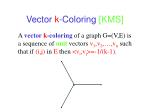### Vector k-Coloring [KMS]

Avector k-coloring of a graph G=(V,E) is a sequence of unit vectors v1,v2,…,vn such that if (i,j) in E then <vi,vj>=-1/(k-1).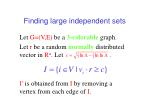### Finding large independent sets

Let G=(V,E) be a 3-colorable graph.

Let r be a random normally distributed vector in Rn. Let .

I’ is obtained from I by removing a vertex from each edge of I.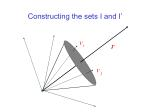### Constructing the sets I and I’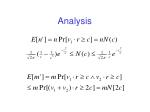### Analysis### Analysis (Cont.)### Analysis (Cont.)### Analysis (Cont.)### A simple observation

Suppose G=(V,E) is k-colorable.

Either G[N(u,v)] is (k-2)-colorable,

or u and v get the same color under

any a k-coloring of G.### A lemma of Blum

Let G=(V,E) be a k-colorable graph with

minimum degree d

for every

Then, it is possible to construct, in polynomial time, a collection {Ti} of about n subsets of V such that at least one Tisatisfies:

|Ti|=W(d2/s)

Tihas an independent subset of size### A lemma of Blum### Graph coloring techniques

Karger Motwani Sudan

Wigderson

Blum

Alon

Kahale

Blum Karger

Our Algorithm### The new algorithm

Step 0:

If k=2, color the graph using 2 colors.

If k=3, color the graph using n3/14 colors using the algorithm of Blum and Karger.### The new algorithm

Step 1:

Repeatedly remove from the graph vertices of degree at most na(k)/(1-2/k). Let U be the set of vertices removed, and W=V-U.

Average degree of G[U] is at most na(k)/(1-2/k).

Minimum degree of G[W] at least na(k)/(1-2/k).

If |U|>n/2, use [KMS] to find an independent set of size n/D1-2/k= n1-a(k).### Step 1

Let d=na(k)/(1-2/k).

Average degree of G[U] is at most d.

Minimum degree of G[W] at least d.### The new algorithm

Step 2:

For every u,v such that N(u,v)>n(1-a(k)/(1-a(k-2)),

apply the algorithm recursively on G[N(u,v)] and k-2.

If G[N(u,v)] is (k-2)-colorable, we get an independent set of size |N(u,v)|1-a(k-2)>n1-a(k).

Otherwise, we can infer*that u and v must be assigned the same color.### The new algorithm

Step 3:If we reach this step then |W|>n/2, the minimum degree of G[W] is at least na(k)/(1-2/k),

and for every u,v in W, N(u,v)>n(1-a(k)/(1-a(k-2)).

By Blum’s lemma, we can find a collection {Ti} of about n subsets of W such that at least one Ti satisfies |Ti|=W(d2/s) and Tihas an independent subset of size .

By the extension of the Alon-Kahale result,

we can find an IS of size### The recurrence relation### Hardness results

It is NP-hard to 4-color 3-colorable graphs [Khanna,Linial,Safra ‘93] [Guruswami,Khanna ‘00]

For any k, it is NP-hard to k-color

2-colorable hypergraphs